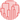# Dendrogram with heat map

When you use a dendrogram to display the result of a cluster analysis, it is a good practice to add the corresponding heatmap. It allows you to visualise the structure of your entities (dendrogram), and to understand if this structure is logical (heatmap). This page aims to describe how to use the `clustermap()` function of seaborn to plot a dendrogram with heatmap. (Note that the seaborn documentation is awesome!)

## Default

You can build a dendrogram and heatmap by using the `clustermap()` function of seaborn library. The following example displays a default plot.

``````# Libraries
import seaborn as sns
import pandas as pd
from matplotlib import pyplot as plt

# Data set
url = 'https://raw.githubusercontent.com/holtzy/The-Python-Graph-Gallery/master/static/data/mtcars.csv'
df = df.set_index('model')

# Default plot
sns.clustermap(df)

# Show the graph
plt.show()``````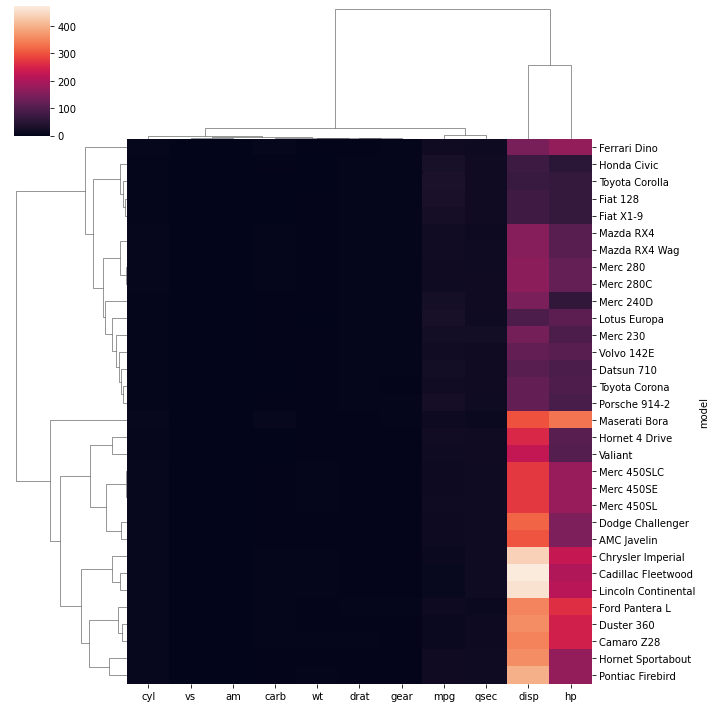## Normalize

It is possible to standardize or normalize the data you want to plot by passing the `standard_scale` or `z_score` aguments to the function:

• `standard_scale` : Either 0 (rows) or 1 (columns)
• `z_score` : Either 0 (rows) or 1 (columns)
``````# Libraries
import seaborn as sns
import pandas as pd
from matplotlib import pyplot as plt

# Data set
url = 'https://raw.githubusercontent.com/holtzy/The-Python-Graph-Gallery/master/static/data/mtcars.csv'
df = df.set_index('model')

# Standardize or Normalize every column in the figure
# Standardize:
sns.clustermap(df, standard_scale=1)
plt.show(
)
# Normalize
sns.clustermap(df, z_score=1)
plt.show()``````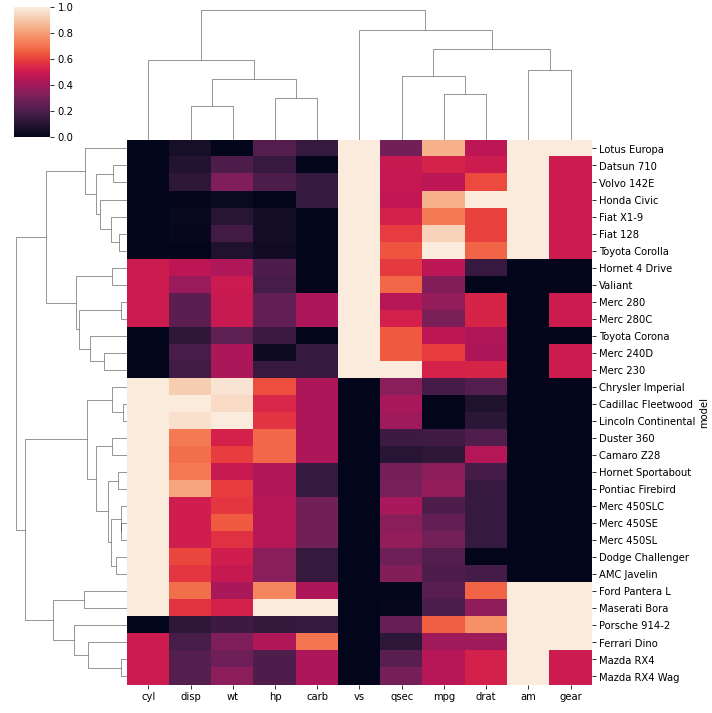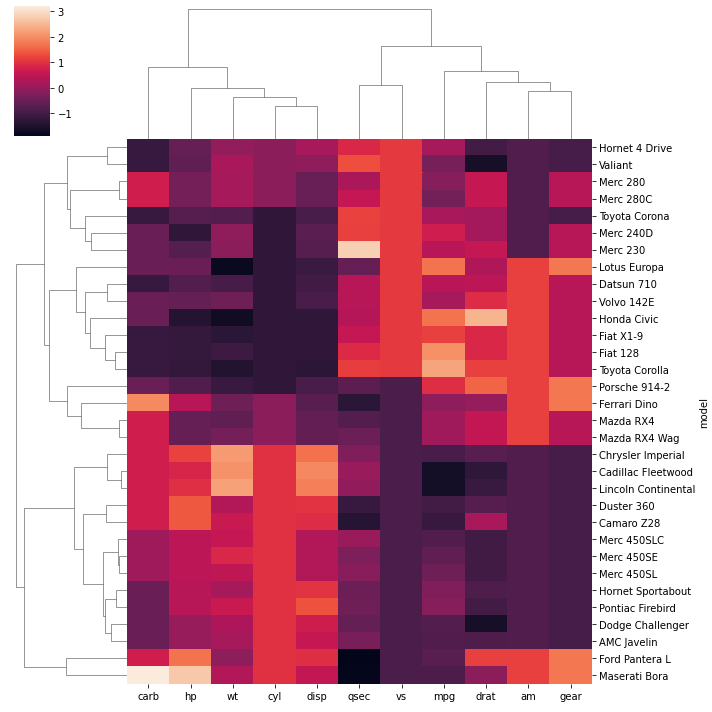## Distance Method

You can use different distance metrics for your data using the `metric` parameter. The most common methods are correlation and euclidean distance.

``````# Libraries
import seaborn as sns
import pandas as pd
from matplotlib import pyplot as plt

# Data set
url = 'https://raw.githubusercontent.com/holtzy/The-Python-Graph-Gallery/master/static/data/mtcars.csv'
df = df.set_index('model')

# plot with correlation distance
sns.clustermap(df, metric="correlation", standard_scale=1)
plt.show()

# plot with euclidean distance
sns.clustermap(df, metric="euclidean", standard_scale=1)
plt.show()``````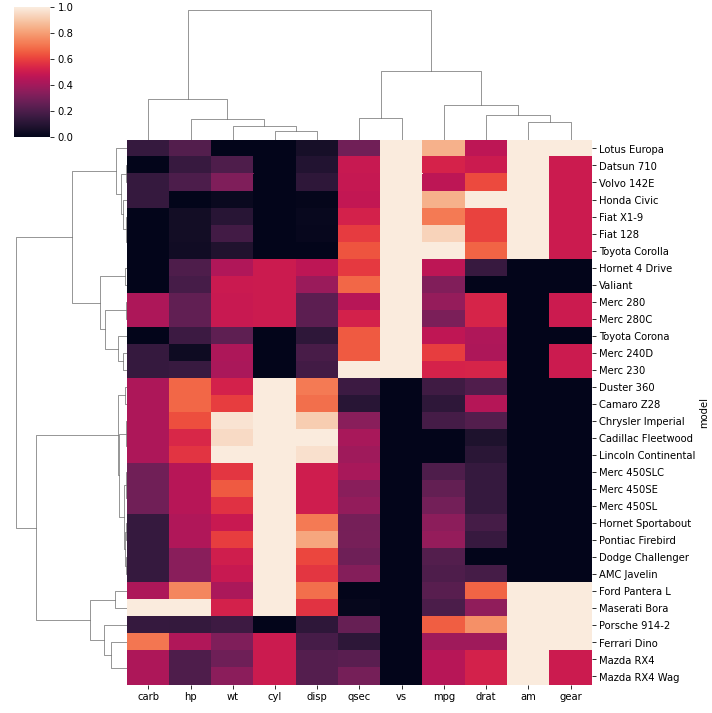## Cluster Method

Since we determined the distance calculation method, now we can set the linkage method to use for calculating clusters with the `method` parameter.

``````# Libraries
import seaborn as sns
import pandas as pd
from matplotlib import pyplot as plt

# Data set
url = 'https://raw.githubusercontent.com/holtzy/The-Python-Graph-Gallery/master/static/data/mtcars.csv'
df = df.set_index('model')

# linkage method to use for calculating clusters: single
sns.clustermap(df, metric="euclidean", standard_scale=1, method="single")
plt.show()

# linkage method to use for calculating clusters: ward
sns.clustermap(df, metric="euclidean", standard_scale=1, method="ward")
plt.show()``````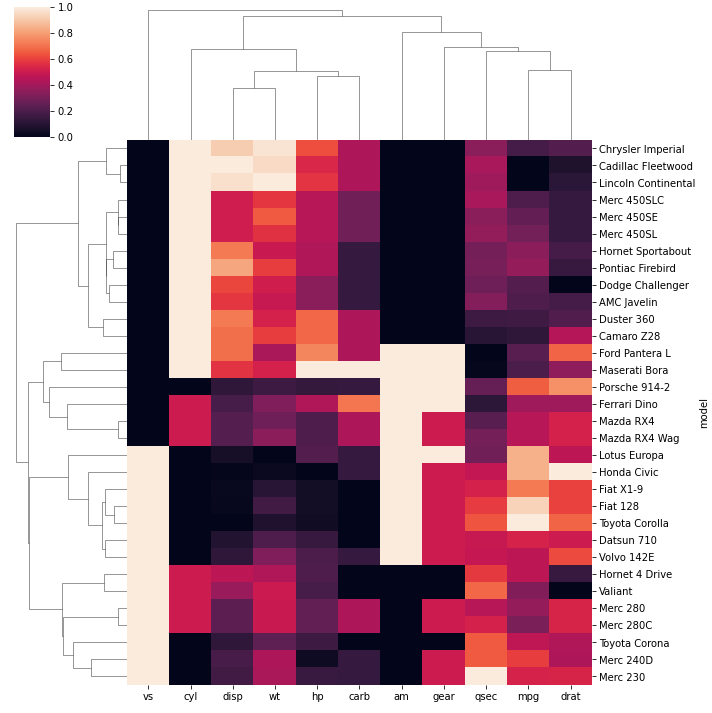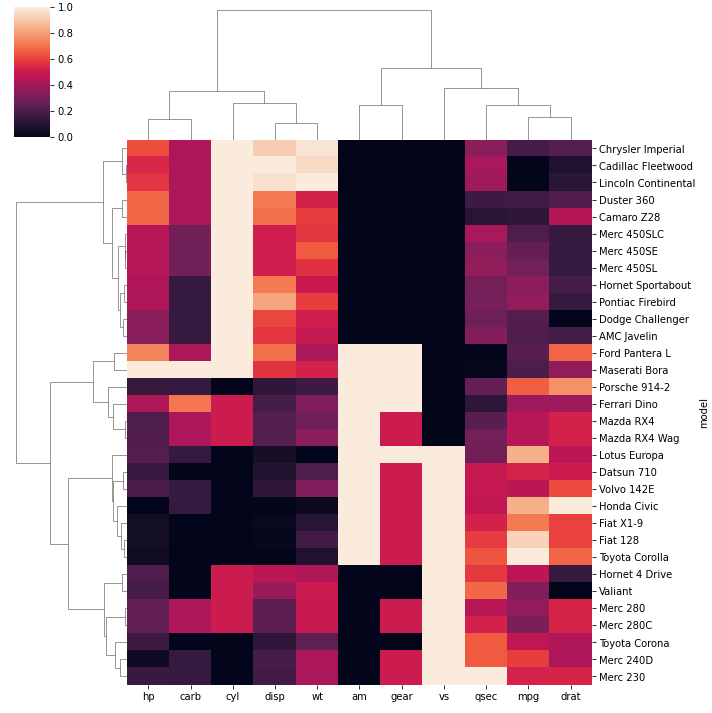## Color

The color palette can be passed to the `clustermap()` function with the `cmap` parameter.

``````# Libraries
import seaborn as sns
import pandas as pd
from matplotlib import pyplot as plt

# Data set
url = 'https://raw.githubusercontent.com/holtzy/The-Python-Graph-Gallery/master/static/data/mtcars.csv'
df = df.set_index('model')

# Change color palette
sns.clustermap(df, metric="euclidean", standard_scale=1, method="ward", cmap="mako")
plt.show()
sns.clustermap(df, metric="euclidean", standard_scale=1, method="ward", cmap="viridis")
plt.show()
sns.clustermap(df, metric="euclidean", standard_scale=1, method="ward", cmap="Blues")
plt.show()``````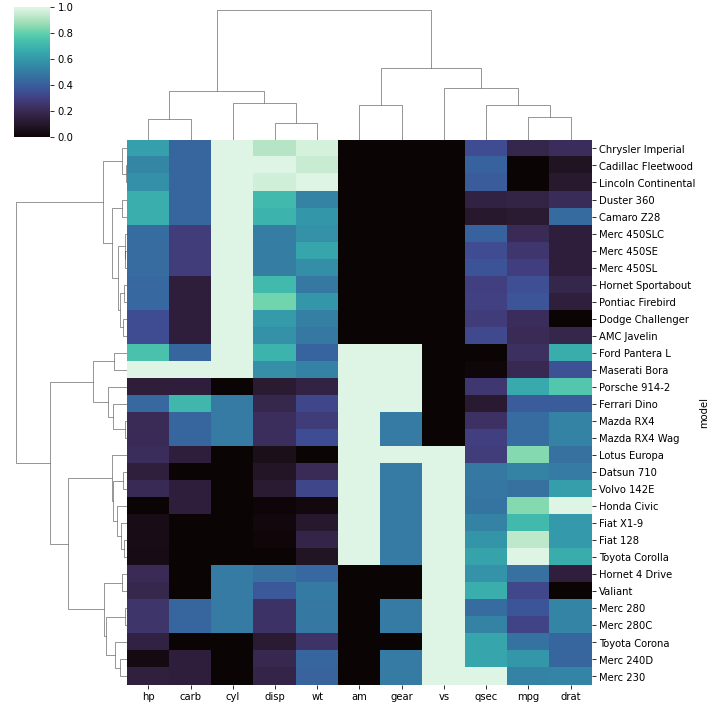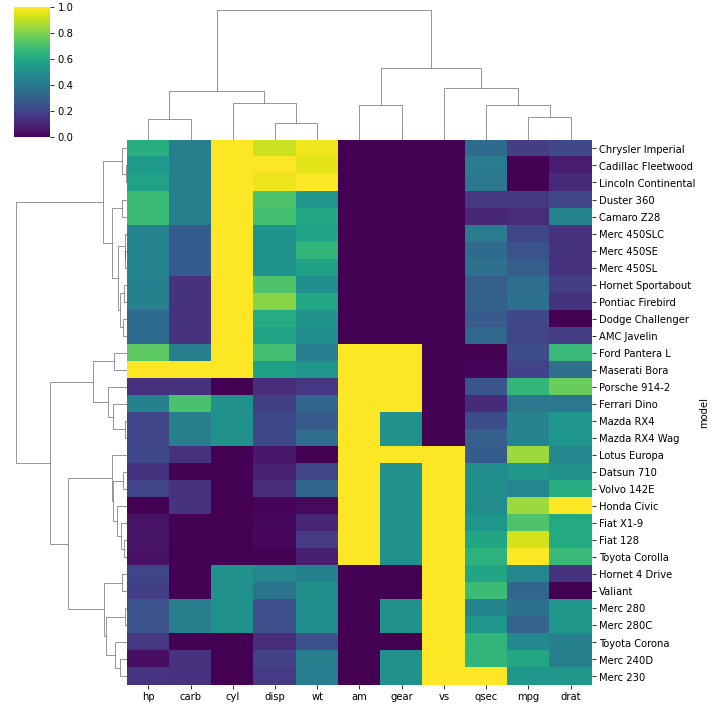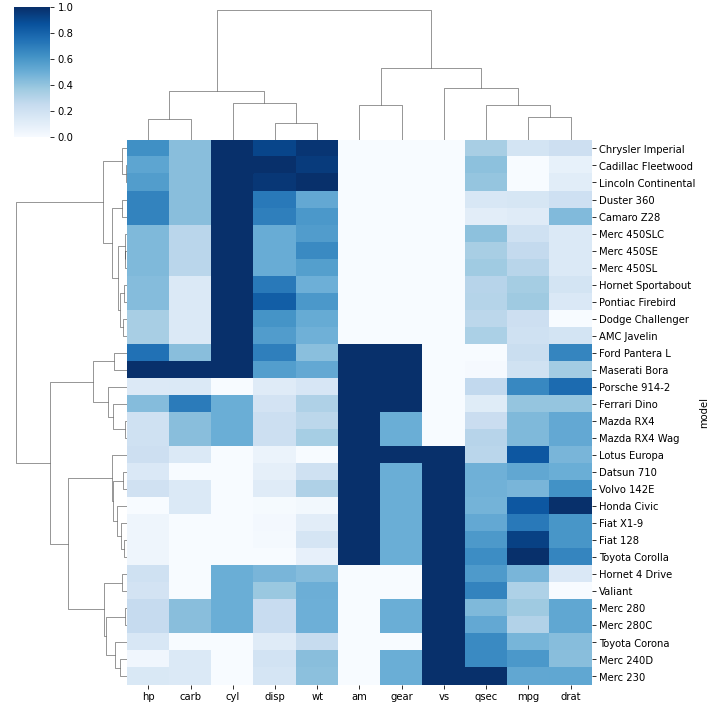## Outliers

In order to ignore an outlier in a heatmap, you can use `robust` parameter:

• `robust` : If True, the colormap range is computed with robust quantiles instead of the extreme values
``````# Libraries
import seaborn as sns
import pandas as pd
from matplotlib import pyplot as plt

# Data set
url = 'https://raw.githubusercontent.com/holtzy/The-Python-Graph-Gallery/master/static/data/mtcars.csv'
df = df.set_index('model')

# Let's create an outlier in the dataset:
df.loc[15:16,'drat'] = 1000

# use the outlier detection
sns.clustermap(df, robust=True)
plt.show()

# do not use it
sns.clustermap(df, robust=False)
plt.show()``````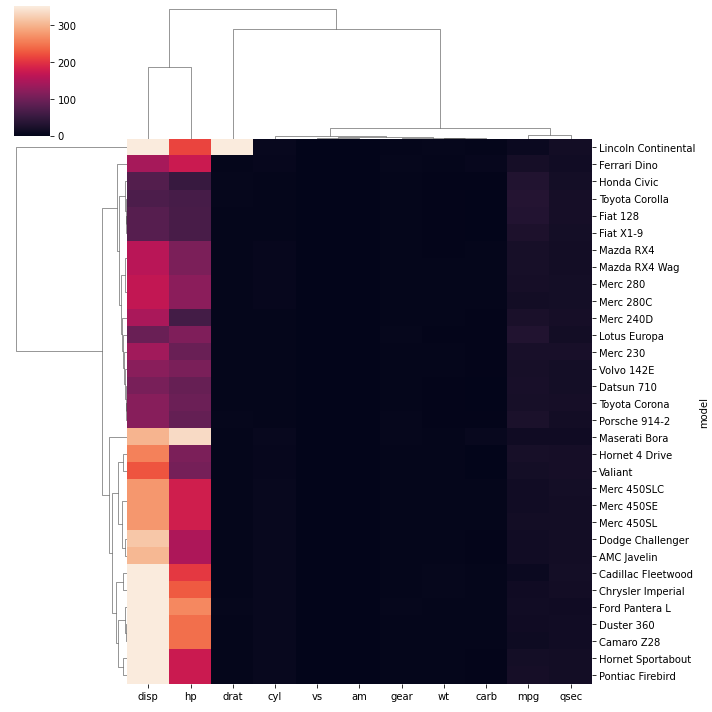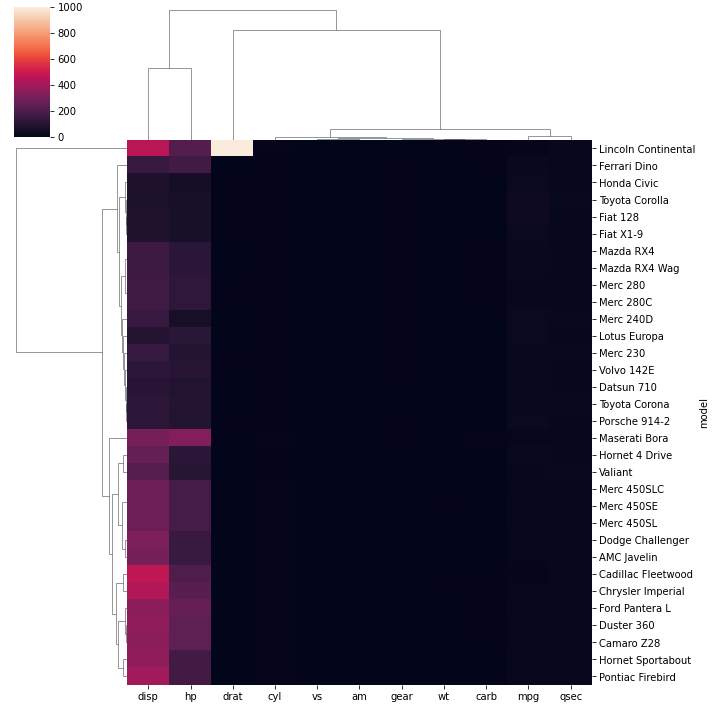## Contact & Edit

👋 This document is a work by Yan Holtz. Any feedback is highly encouraged. You can fill an issue on Github, drop me a message onTwitter, or send an email pasting `yan.holtz.data` with `gmail.com`.